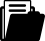Meine Position
i
Ein Tipp vom Eldar Team
Je mehr Sie bestellen, desto grösser Ihr RabattBuch
Buch
Fachbuch
2010

# Infinite Regress Arguments

ISBN
EAN
978-90-481-3340-6
9789048133406
Artikel-Nr.
5EZ3PV6
Bestpreis-Garantie
Kostenloser Versand
Rabatt
-22.5
%
CHF 179.00
CHF
138.73
Anzahl
1
Maximale
Lieferzeit
33
Arbeitstage
Montag
08.11.2021Beschreibung

Infinite regress arguments are part of a philosopher's tool kit of argumentation. But how sharp or strong is this tool? How effectively is it used? The typical presentation of infinite regress arguments throughout history is so succinct and has so many gaps that it is often unclear how an infinite regress is derived, and why an infinite regress is logically problematic, and as a result, it is often difficult to evaluate infinite regress arguments. These consequences of our customary way of using this tool indicate that there is a need for a theory to re-orient our practice.

My general approach to contribute to such a theory, consists of collecting and evaluating as many infinite regress arguments as possible, comparing and contrasting many of the formal and non-formal properties, looking for recurring patterns, and identifying the properties that appeared essential to those patterns. Two very general questions guided this work: (1) How are infinite regresses generated in infinite regress arguments? (2) How do infinite regresses logically function as premises in an argument? In answering these questions I clarify the notion of an infinite regress; identify different logical forms of infinite regresses; describe different kinds of infinite regress arguments; distinguish the rhetoric from the logic in infinite regress arguments; and suggest ways of improving our discussion and our practice of constructing and evaluating these arguments.

Inhaltsverzeichnis
Introduction.- Chapter 1 What is an infinite regress argument? 1.1 The general structure of infinite regress arguments. 1.2 Boundaries of an infinite regress argument. 1.2.1 Boundaries when an infinite regress is vicious. 1.2.2 Boundaries when an infinite regress is benign. 1.3 A hypothesis H about the nature of infinite regresses. 1.4 Testing hypothesis H. 1.5 Testing hypothesis H with nonconcatenating regresses. 1.6 Other features of infinite regresses. 1.7 The necessary quantity of terms and relations. 1.8 The relation of terms and objects of infinite regresses. 1.9 Applications of hypothesis H to various example. 1.9.1 Plato’s couch. 1.9.2 Teachers taught by teachers. 1.9.3 Gods giving meaning to Gods. 1.9.4 Maps of maps. 1.9.5 Lewis Carroll’s 'What the Tortoise said to Achilles'. 1.10 Logical functions of infinite regresses. 1.10.1 Benign regresses. 1.10.2 Superfluous regresses. 1.11 Cogency and benign regresses. Chapter 2 Formal and nonformal logic of infinite concatenating regresses. 2.1 Recurring terms, loops, and regress formulas. 2.2 Applications. 2.3 Recurring terms, loops, and infinite concatenating regresses. 2.4 Relations and loops. 2.5 Blocking all possible loops. 2.6 Are irreflexivity, or asymmetry or transitivity necessary to block loops? 2.7 Concatenating relations in regress formulas. 2.8 Directions of infinite concatenating regresses. 2.9 Non-formal considerations in regress formulas. 2.9.1 Relations and their implications. 2.9.2 Stated properties of terms. 2.9.3 Unstated properties of terms. 2.10 Summary. 2.11 Evaluative questions. Chapter 3 Viciousness. 3.1 Are there inherently vicious regresses? 3.2 Clark on viciousness. 3.3 Johnstone and viciousness. 3.4 Uncompletability and viciousness. 3.5 Occam's Razor: ontological extravagance. 3.6 Blocking some vicious regresses. 3.6.1 Hume. 3.6.2 Miller. 3.6.3 Laurence and Margolis. 3.6.4 The general form of the argument for blocking regresses.Chapter 4 Circular definitions and circular explanations. 4.1 A formal derivation of infinite regresses from circular definitions. 4.2 Infinitely many infinite regresses. 4.3 Semantic considerations. 4.4 Regresses independent of circularity. 4.5 The viciousness of infinite regresses entailed by circular definitions. The derivation of infinite regresses from circular explanations. Chapter 5 Infinite regresses of recurring questions. 5.1 Recurring questions and the derivation of infinite regresses. 5.2 Recurring questions and vicious regresses. Chapter 6 Infinite regresses of recurring problems and responses. 6.1 Plato’s aviary in the Theatetus. 6.2 McTaggart's discontinual regress. 6.3 Mackie's discontinual regress. 6.4 Armstrong’s continual regress. 6.5 A continual regress in a version of Cantor’s diagonal method. 6.6 Lehrer’s non-continual regress. 6.7 Evaluative questions.Herausgeber/-in
Zielgruppe
Research
Leserkritik

From the reviews:

“This is a much welcomed book. Its main message is: make your infinite regress argument explicit! The typical presentation of such an argument is, as Gratton puts it, ‘so succinct and has so many gaps’ … that we hardly know what to think of it. … The book is a very welcome addition to the philosophical toolkit.”­­ (Jan Willem Wieland, Argumentation, Vol. 25, 2011)
Publikation
Niederlande
04.02.2010Sprache
EnglischFormat
Hardcover
211 SeitenWeitere FormateVersand
Kostenloser Versand: Schweiz & Liechtenstein
Für den Versand nach Deutschland oder Frankreich werden die Versandgebühren der Schweizerischen Post berechnet. Diese werden Ihnen im Warenkorb für Ihre gesamte Bestellung berechnet.Themen
Zurück
Zum Start